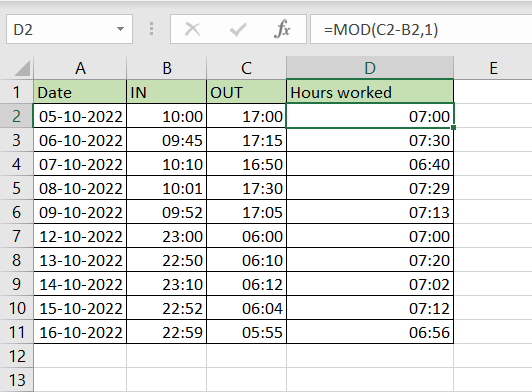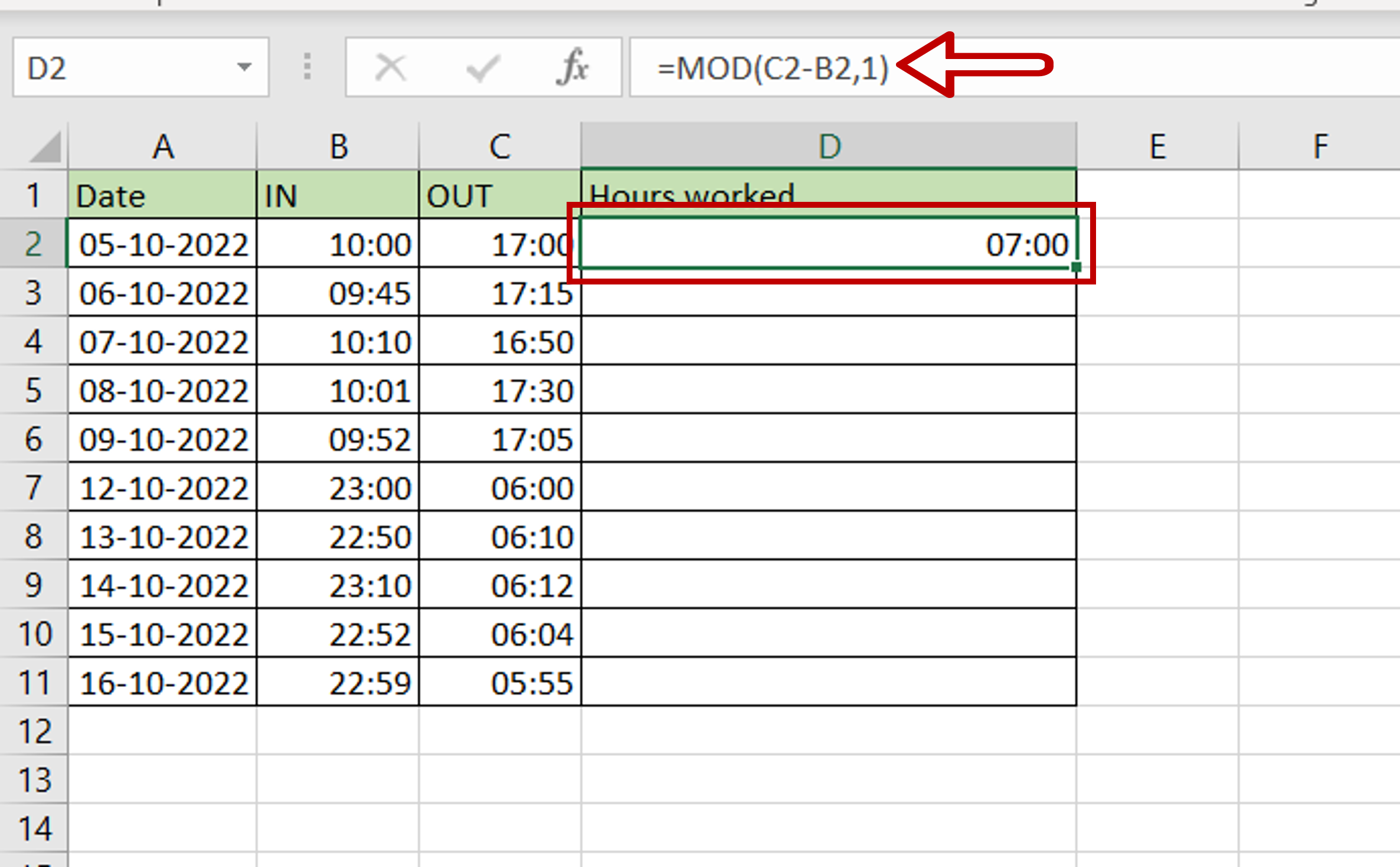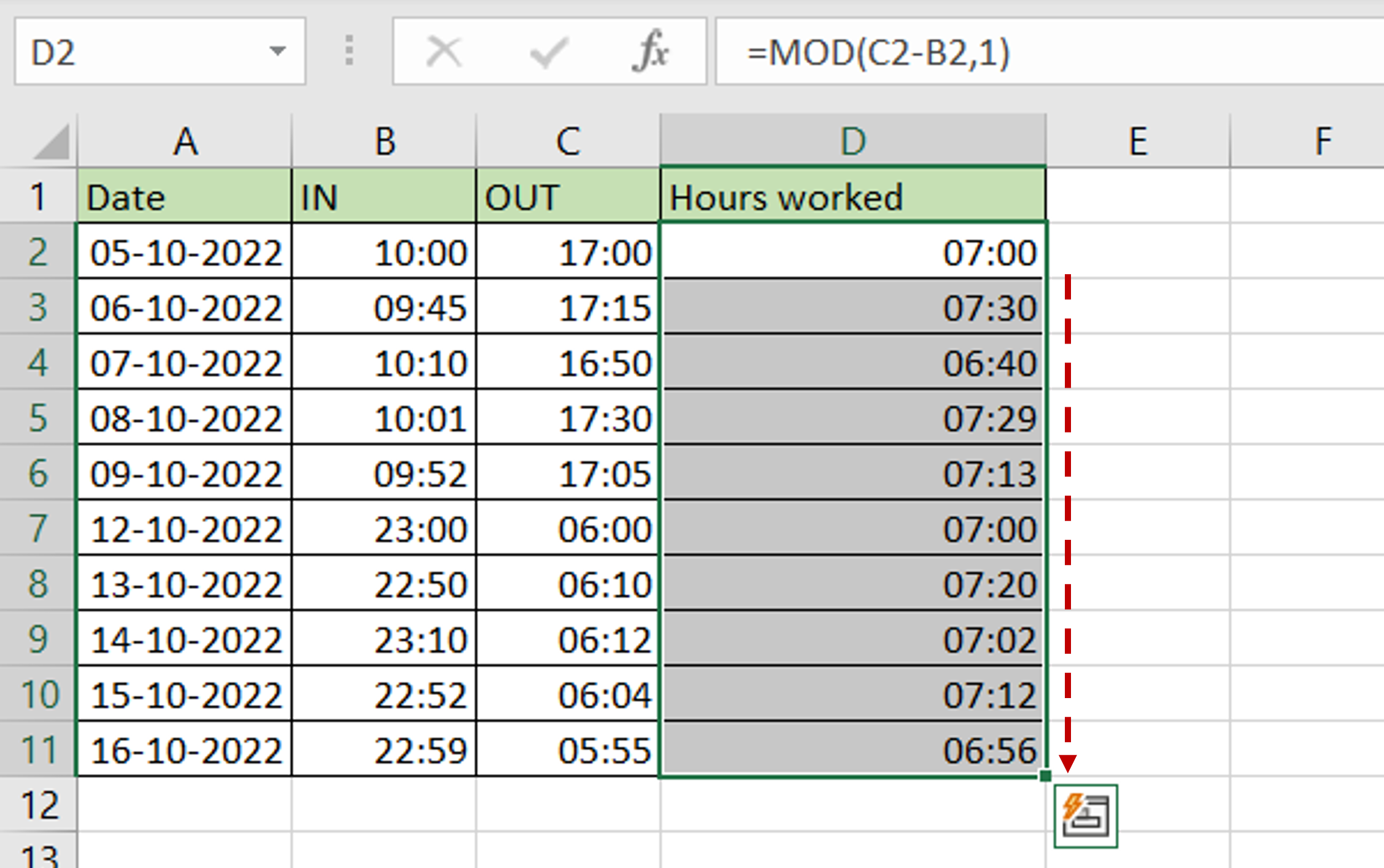# How to calculate hours worked in Excel using a 24-hour clock

You can watch a video tutorial here.

Assume you have an employee who works both day shifts and night shifts. To get the hours worked for the day shift, simple subtraction between the IN and OUT times will give you the result. For night shifts, simple subtraction will not work because the OUT time is lesser than the IN time as it is on the next day. To create a formula that will work in both scenarios, we adapt the MOD() function.

1. MOD() function: this returns the remainder of a number that is divided by a divisor
1. Syntax: MOD(value, divisor)
1. value: the number to be divided
2. divisor: the number by which the value will be divided

In this example, we will use the MOD() function with a divisor of 1 to get rid of the negative number.Excel treats date and time formats differently from numbers and text. Because of the different scales i.e. 24 hours, 60 minutes, and 60 seconds, when adding or subtracting minutes and seconds they cannot be treated like regular numbers. To calculate hours worked, you need to find the difference between 2 times.  In Excel, to be able to add or subtract times, you need to format the value as time for the operation to work correctly.

### Step 1 – Create the formula– Select the cell where the result is to appear
– Type the formula using cell references:
=MOD(OUT-IN,1)
– Press Enter

### Step 2 – Copy the formula– Using the fill handle from the first cell, drag the formula to the remaining cells
OR
a) Select the cell with the formula and press Ctrl+C or choose Copy from the context menu (right-click)
b) Select the rest of the cells in the column and press Ctrl+V or choose Paste from the context menu (right-click)